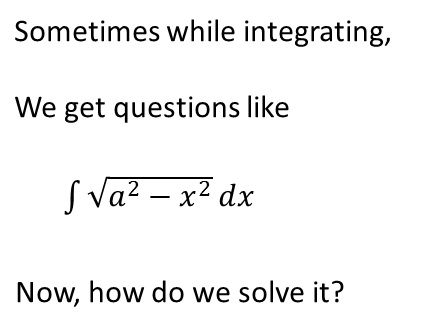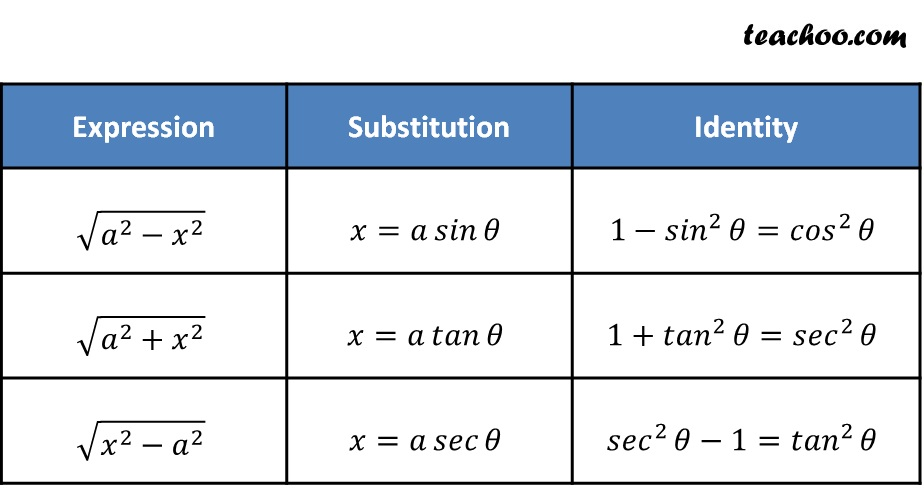Not clear how to approach

Chapter 2 Class 12 Inverse Trigonometric Functions
Concept wiseThere are some standard substitutions, where we put value of x and solve itLearn in your speed, with individual attention - Teachoo Maths 1-on-1 Class

### Transcript

Sometimes while integrating, We get questions like ∫1▒√(𝑎^2−𝑥^2 ) 𝑑𝑥 Now, how do we solve it? There are some standard substitutions, where we put value of x and solve it √(𝑎^2−𝑥^2 ) 𝑥=𝑎 𝑠𝑖𝑛⁡𝜃 1−〖𝑠𝑖𝑛〗^2⁡𝜃=〖𝑐𝑜𝑠〗^2⁡𝜃 √(𝑎^2+𝑥^2 ) 𝑥=𝑎 𝑡𝑎𝑛⁡𝜃 1+〖𝑡𝑎𝑛〗^2⁡𝜃=〖𝑠𝑒𝑐〗^2⁡𝜃 √(𝑥^2−𝑎^2 ) 𝑥=𝑎 𝑠𝑒𝑐⁡𝜃 〖𝑠𝑒𝑐〗^2⁡𝜃−1=〖𝑡𝑎𝑛〗^2⁡𝜃